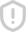### Python实现C语言经典100例 评分fi<=10: onus 冲0.1 print bonus lif 20 bonus bonusl-(i-10)*0.075 print bonus elif i<=40: bonus= bonus2+(i-20)*0.05 lif i<- 60 bonus bonus +(i-40)* 0.03 lif i<-100 bonus= bonus +(i-60) *0.015 e⊥Se bonus-bonus10 +(i-100)*0.01 print bonus = bonus 程序3】 题目:一个整数,它加上100后是一个完全平方数,再加上168又是一个完全平方数,请 问该数是多少? 1.程序分析:在10万以内判断,先将该数加上100后再开方,共将该数加上268后再开 方,如果开方后 的结果满足如下条件,即是结果。请看只体分析: 2.程序源代码: #include math. h ) long int for(i=1;i<100000:i++) x=Sgrt(i+100) /*ⅹ为加上100后开方后的结果* y-sqrt(i+268);*y为再加上168后开方后的结果* 来如果一个数的半方根的平方等于该数,这说明此数是完全平方数来 printf( \n%1d\n", i) import math for i in range (10000) 转化为整型值 x- int(math. sgrt(i+100)) y= int(math. sqrt(iI 268)) i-100)and( rint 1 我的程厅 if(1-0): import math for e in range(10000 if (int(math. sart(e+100))*int(math. sart(c+100 e+100) f(int(math. sqrt(e+268))*int(math. sgrt print e 程序4】 题目:输入某年某月某日,判新这一天是这一年的第几天? 1.程序分析:以3月5日为例,应该先把前两个月的加起来,然后再加上5天即本年的第几 天,特殊 情况,闰年且输入月份大于3时需考虑多加天。 2.程序源代码: year= int(raw input( year: n') month int (raw input(month: n')) day= int (raw input(day: \n')) months=(0,31,59,90,120,151,181,212,243,273,304,334) if 0<= month <=12 sum= months [nonth-11 print data erro sum +-day leap=0 f (year% 400==0) or((year %4=0) and (year 100! =0) leap- 1 f (leap = 1) and(month >2) sum +=1 print it is the %odth day. % sum 程序5】 趣日:输入三个整数x,y,z,请把这三个数由小到大输出。 1.程序分析:我们想小法把最小的数放到x上,先将x与y进行比较,如果x>y则将ⅹ与y 的值 进行交换, 然后再用x与z进行比较,如果x》z则将ⅹ与z的值进行交换,这样能使x最 小 2.程序源代码: for i in range (3) ⅹ=int( raw input( integer:Ⅶn)) 1. append (x) 1. sort(#川序排序 print 1 程序6】 题目:用*号输出字母C的图案。 1.程序分析:可先用*号在纸上写出字封C,再分行输出。 2.程序源代码 print Hello Py thon world! \n print’米*10 print* rint’**10 print米\n3*6 程序7】 题目:输出特殊图案,请在c环境中运行,看一看, Very beautiful! 1.程序分析:字符共有256个。不同字符,图形不一样。 2.程序源代码 6 b=219 print chr(b), chr(a), chr(a), chr(a), chr(b) print chr(a), chr(b), chr(a), chr(b), chr(a) print chr(a), chr(a), chr(b), chr(a), chr(a) print chr(a), chr(b), chr(a), chr(b), chr(a) int chr(h chr(a, ch (a), chr(a), chr(b) 程序8】 题目:输出9*9口诀。 1.程序分析:分行与列考虐,共9行9列,i控制行,j控制列。 2.程序源代码 #include stdio. h main int i, j, result; printf( \n") for(i=1;i<10;i+-) for(j=1;j<10;j+) result=i printf("%d水%d--3d",i,j, result);/来3d表小左对齐,占3位* ("n");/每一行后换行 f (1,10) for j in range(1, 10): result print’%d米%d=%3d’%(i,j, result) print 程序9】 趣目:要求输出国际象棋棋盘 1.程序分析:用i控制行,j来控制列,根据i+j的和的变化来控制输出黑方格,还是白方 格 2.程序源代码 #include stdio. h Ⅲlr int 1, j for(i-0;i<8;i++) for(j=0;j<8;j++) f(i+j)%2--0) rinf("%c%c",219,219) e⊥se printf(a) printf(\n") aport sys for i in range(8 for j in range ( 8) if(i+j)%2==0: sys. stdout. write(chr (219)) sys. stdout. write(chr(219)) sys. stdout. write(’) ￥int 我的程序 Import sys for i in range(1, 10) range(1, i+1) sys. stdout. write(%d *s %d=%-3d%(j, i, i*j)) sys. stdout. write( print 程序10】 题目:打印楼梯,同时在楼梯上方打印两个笑脸。 1.程序分析:用i控制行,j来控制列,j根据i的变化来控制输出黑方格的个数。 2.程序源代码 t sys. stdout. write(chr(1)) sys. stdout. write(chr(1)) int for i in range(1, 11) j in range (1, i) sys. stdout. write(chr(219)) sys. stdout. write(chr(219)) print 程序11】 题目:古典问题:有一对兔子,从出生后第3个月起每个月都生一对兔子,小兔子长到第 个月 后每个月又生一对兔子,假如兔子都不死,问每个月的兔子总数为多少? 1.程序分析:兔子的规律为数列1,1,2,3,5,8,13,21 2.程序源代码: main long fl, f2 int 1 for(i=1;i<=20;i++) printf(%12ld%12ld", fl, f2) if(i%2-0) printf("Ⅶn";/*控制输出,每行四个 f1=f1+f2;/*前两个月加起来赋值给第三个月* f2=1+r2;/*前两个月加起来赋值给第三个月 2=1 for i in range(1, 21) print’%12d%12d'’%(f1,f2) if(i%2)-0 rint fl fl + f2 f2-f1+f2 程序12】 遨日:判断101-200之间有多少个素数,并输出所有素数。 1.程序分析:判断素数的方法:用一个数分别去除2到sqrt(这个数),如果能被整除, 则表明此数不是素数,反之是素数。 2.程序源代码 h=0 leap=1 from math import sgrt from sys import stdout for m in range(101, 201) k= int(sgrt(m +1)) for i in range(2, k+1) f m break print’%4d’%m ifh%10-0 int leap-1 nt’ The total is%d’%h 我的程序 import sys import math def TsPrim(n return Truc nt(math. sgrt(n)) range(3, n) fn‰ return False return true naime mmain i-0 for e in range(101, 201) f IsPrim(e sys. stdout. writ fi%5=-0 print 程序13】 题目:打印出所有的“水仙花数”,所谓“水仙化数”是指一个三位数,其各位数字立方和 等 于该数 本身。例如:153是个“水仙花数”,因为153=1的三次方+5的三次方+3的三次 方 1.程序分析:利用for循环控制100999个数,每个数分解出个位,十位,百位。 2.程序源代码 for n in range(100, 1001) i-n/100 j=n/10%10 k=n%10 ifi*100+j*10+k=i+j*2+k收3: print%-5d gc 我的程序 if1--1 for x in range(1, 10) for y in range(0, 10) for z in range(0, 10) m=x*1001y*101z if(x来3+y来3z3—m): rint e⊥se for n in range(100, 1000) i=n/100 /10%10 print 1, j,k f n==1**3+j**3+k**3: int% 5d"% 程序14】 趣目:将一个正整数分解质因数。例如:输入90,打印出90-2*3米3*5 程序分析:对n进行分解质因数,应先找到一个最小的质数k,然后按下述步骤完成: (1)如果这个质数恰等」n,则说明分解质因数的过程己经结束,打印出即可。 (2)如果n<k,但n能被k整除,则应打印出k的值,并用n除以k的商,作为新的正整数你 重复执行第一步 (3)如果n不能被k整除,则用k-1作为k的值,重复执行第一步。 2.程序源代码: from sys import stdout n= int(raw input( input number: \n )) print n=%d %n for i in range(2, n 1) e n f n o

...展开详情举报收藏分享ModbusTCP／RTU网关设计 立即下载html+css+js制作的一个动态的新年贺卡 立即下载iCopy解码软件v1.0.1.7.exe 立即下载Camtasia 9安装及破解方法绝对有效 立即下载source insight 4.0.0087 注册机序列号Patched(2017/10/17) 立即下载Java项目经验汇总(简历项目素材） 立即下载C++ Primer 中文版（第 5 版） 高清无水印 pdf 立即下载rainmeter雨滴皮肤合集(30个） 立即下载C#学生信息管理系统 立即下载wifi密码字典包1G 立即下载rswofxd